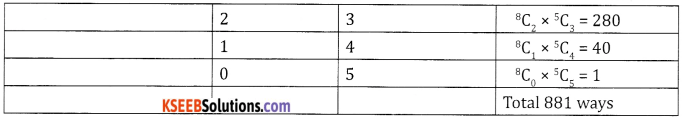# 2nd PUC Basic Maths Question Bank Chapter 2 Permutations and Combinations Ex 2.3

Students can Download Basic Maths Exercise 2.3 Questions and Answers, Notes Pdf, 2nd PUC Basic Maths Question Bank with Answers helps you to revise the complete Karnataka State Board Syllabus and score more marks in your examinations.

## Karnataka 2nd PUC Basic Maths Question Bank Chapter 2 Permutations and Combinations Ex 2.3

Part – A

2nd PUC Basic Maths Permutations and Combinations Ex 2.3 One Mark Questions and Answers

Question 1.
In how many ways a committee of 5 can be chosen from 10 students.
10C5 ways = 252 ways,

Question 2.
Find n if  nC10 = nC15
Given nC10 = nC15 ⇒ n = 10 + 15 = 25

Question 3.
Find x if  9Cx + 9C7 = 10C7
Given 9Cx + 9C7 = 10C7
w.k.t nCr + nCr-1 = n+1Cr
∴ x = 8

Question 4.
How many straight lines can be formed from 10 points if no three of them are collinear.
10C2 ways = 45 ways

Question 5.
How many triangles can be formed from 8 non collinear points.
8C3 ways = 56 ways.Part – B

2nd PUC Basic Maths Permutations and Combinations Ex 2.3 Two Marks Questions and Answers

Question 1.
If nC8 = nC12 find nC5.
Given nC8 = nC12
⇒ n = 8 + 12 = 20
nC5 = 20C5 = 15,504.

Question 2.
If nPr = 30240 nCr = 252 find r
If nPr = 30, 240, nCr = 252
w.k.t. $$^{n} C_{r}=\frac{^{n} P_{r}}{r !} \Rightarrow r !=\frac{^{n} P_{r}}{^{n} C_{r}}=\frac{30,240}{252}$$
r! = 120
r! = 5!
⇒ r = 5

Question 3.
Find the number of ways in which a committee of 4 Students and 2 Lecturers can be chosen out of 10 students and 8 lecturers.
4 students out of 10 and 2 lecturers out of 8 can be selected in 10C4 × 8C2, ways = 210 × 28 = 5,880

Question 4.
Find r if  25Cr+3 = 25Cr+3
25Cr+325Cr+3 ⇒ r + 3 = 2r – 3 ⇒ r = 6.Question 5.
Find the number of straight lines and triangles that can be formed out of 20 points of which 8 are collinear.
Number of straight lines =
20C28C2 + 1 = 190 – 28 + 1 = 163 straight lines.
Number of triangles = 20C38C3 = 1140 – 56 = 1084.

Question 6.
Find the number of parallelograms that can be formed from a set of 6 parallel lines intersecting another set of 4 parallel lines.
This can be selected in 6C2 × 4C2 = 15 × 6 ways = 90 ways.

Question 7.
Find the number of diagonals of a polygon of 20 sides.
Number of diagonals = nC2 – n = 20C2 – 20 = 190 – 20 = 170 ways.

Question 8.
In a party each person shakes hands with everyone else. If there are 25 members in the party, Calculate the number of handshakes.
Number of hand shakes = 25C2 = $$\frac{25 \times 24}{2 \times 1}$$ = 300 ways

Question 9.
In how many ways can 6 red and 4 white marbles be chosen from a bag containing 10 Red and 6 White marbles.
We have to select 6 marbles out of 10 and 4 white out of 6 white marbles = 10C6 × 6C4 = 210 × 15 = 3,150.

Question 10.
In how many ways can 6 people be chosen out of 10 people if one particular person is always included.
One particular person is included, we have to choose from 9, this can be done in 9C3 ways.Part – C

2nd PUC Basic Maths Permutations and Combinations Ex 2.3 Three marks Questions and Answers

Question 1.
If a convex polygon has 170 diagonals. Find the number of sides of the polygon.
Number of diagonals = nC2 – n = 170.
$$\frac{n(n-1)}{2}-n=170$$
n2 – n – 2n = 340 ⇒ x2 – 3x – 340
(n + 17) = 0
n = 20 or -17
∵ n can’t be negative n = 20

Question 2.
A team of conveners consisting of 4 lecturers, 4 boys and 2 girls has to be selected from 15 lecturers, 10 boys and 12 girls. In how many ways the selection can be made if the 2 lecturers who were conveners last year have to be included and 3 boys who failed in the examination cannot be chosen.∴ Number of selections = 13C2, × 7C4 × 12C2
= 78 × 35 × 66
= 1,80,180.

Question 3.
A committee of 4 has to be chosen from 10 boys and 8 girls. In how many ways can this be done if girls are in a majority.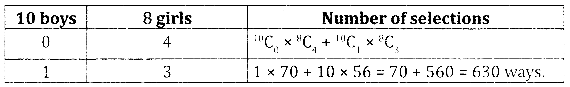Question 4.
If nP5 = 24 ×nC4 find n
Given nP5 = 24 × nC4n – 4 = 1
⇒ n = 4 + 1 = 5

Question 5.
If  npr = 240, nCr = 120 find n and r.
Given npr = 240, nCr = 120
w.k.t ncr = $$\frac{^{n} P_{r}}{r !}$$
∴ $$r !=\frac{240}{120}=2$$
r! = 2! ⇒ r= 2.

Question 6.
A team of 8 players has to be selected from 14 players. In how many ways the selections can be made if
(a) Two particular players are always included.
(b) Two particular players are always excluded.
(a) Two players are always included: We have to choose 6 from 12 players. This can be done in 12C6 ways = 924 ways.
(b) Two players are always excluded: We have to choose 8 from 12 players. This can be done in 12C8 ways = 495 ways.Part – D

2nd PUC Basic Maths Permutations and Combinations Ex 2.3 Five marks Questions and Answers

Question 1.
From a class of 9 boys and 7 girls, 12 students are to be chosen for a competition which includes atleast 6 boys and atleast 4 girls. In how many ways can this be done if a particular boy is always chosen.
There are 9 boys and 7 girls, 12 students are to be chosen which includes atleast 6 boys & 4 girls one particular boy is always chosen ∴ we have to choose atleast 5 boys and 4 girls from 8 boys and 7 girls.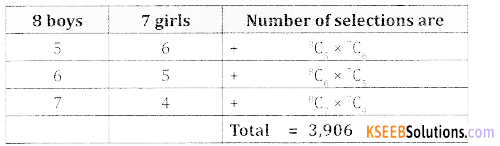Question 2.
A man has 10 relatives, 4 of them are ladies 3 gentlemen and 3 children. In how many ways can he invite 7 relatives to a dinner party so that
(1) there are exactly 2 ladies, 3 gentlemen and 2 children.
(2) there are exactly 2 gentlemen and atleast 3 ladies. (1)
1.Number of selections = 4C2 × 3C3 × 3C2 = 6 × 1 × 3 = 18 ways

(2)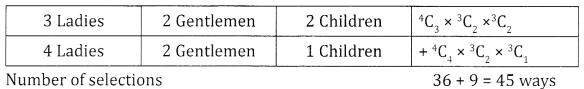Question 3.
From a class of 12 boys and 10 girls, 10 students are to be chosen for a competition, including atleast 4 boys and atleast 4 girls. The 2 girls who won the prize last year should be included. In how many ways can the selections be made.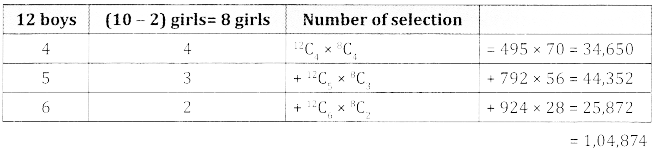Question 4.
Abox contains 5 red, 4 black and 3 white balls. How many selections of 8 balls can be made if the selection contains.
(1) Exactly 4 red, 2 black and 2 white balls.
(2) Atleast 3 red, atleast 3 black and atleast 1 white ball.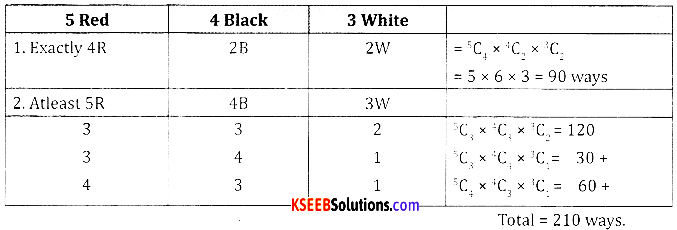Question 5.
An examination paper consists of 12 questions divided into parts A and B contains 7 questions in part A and part B contains 5 questions. A candidate is required to answer 8 questions selecting atleast 3 from each part. In how many ways can the candidate select the questions.Question 6.
A man has 10 relatives, 4 of them all ladies, 3 gentlemen and 3 children. In how many ways can he invite 17 relatives to dinner party so that
(i) there are exactly 2 ladies, 3 gentlemen and 2 children
(ii) there are exactly 2 gentlemen and atleast 3 ladies
(iii) there are exactly 3 children, atleast 1 lady and atleast 2 gentlemen.Question 7.
A team of eleven is to be chosen out of 16 cricket players of whom 4 are bowlers and 2 wicket keepers. In how many ways can the team be chosen so that
(i) there are exactly 3 bowlers and one wicket keeper
(ii) there are atleast 3 bowlers and atleast one wicket keeper.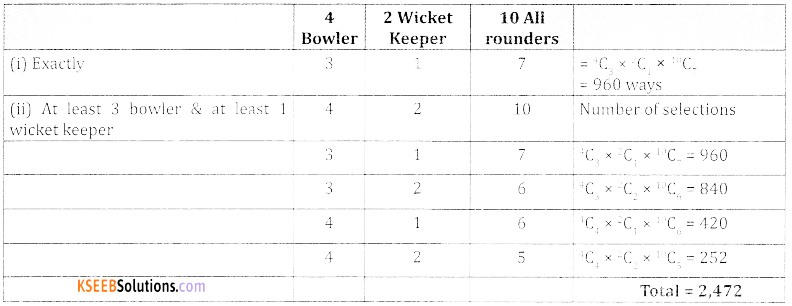Question 8.
Out of 4 officers and 10 clerks in an office a committee consisting of 2 officers and 3 clerks is to be formed. In how many ways can this be done if
(í) any officer and any clerk can be included
(ii) one particular clerk must be on committee
(iii) one particular officer cannot be on the committee.
(i)(ii)
One particular clerk is already on the committee.
∴ There are 2 clerks to be chosen from the remaining 9 clerks can be done in 4C2 × 9C2 = 6 × 36 = 216 ways.

(iii) One particular officer can not be on the committee. We have to choose 2 officers from 3 officers, can be done in 3C2 × 10C3 = 3 × 120 = 360 ways.Question 9.
A committee of 5 are to be formed from 8 Americans and 5 Anglo Indians. In how many ways can this be done when the committee contains
(i) exactly two Anglo – Indians
(ii) atleast two anglo Indians.